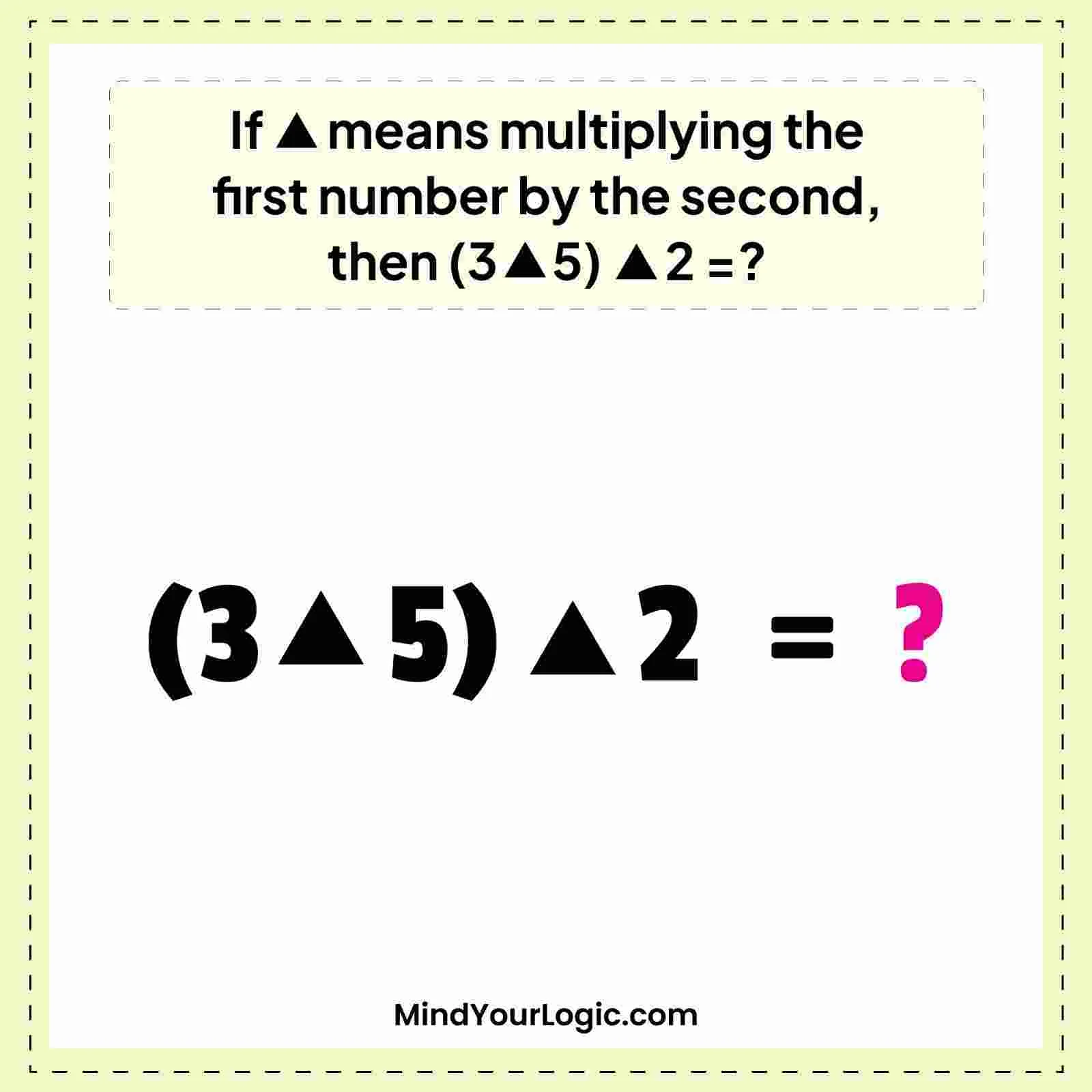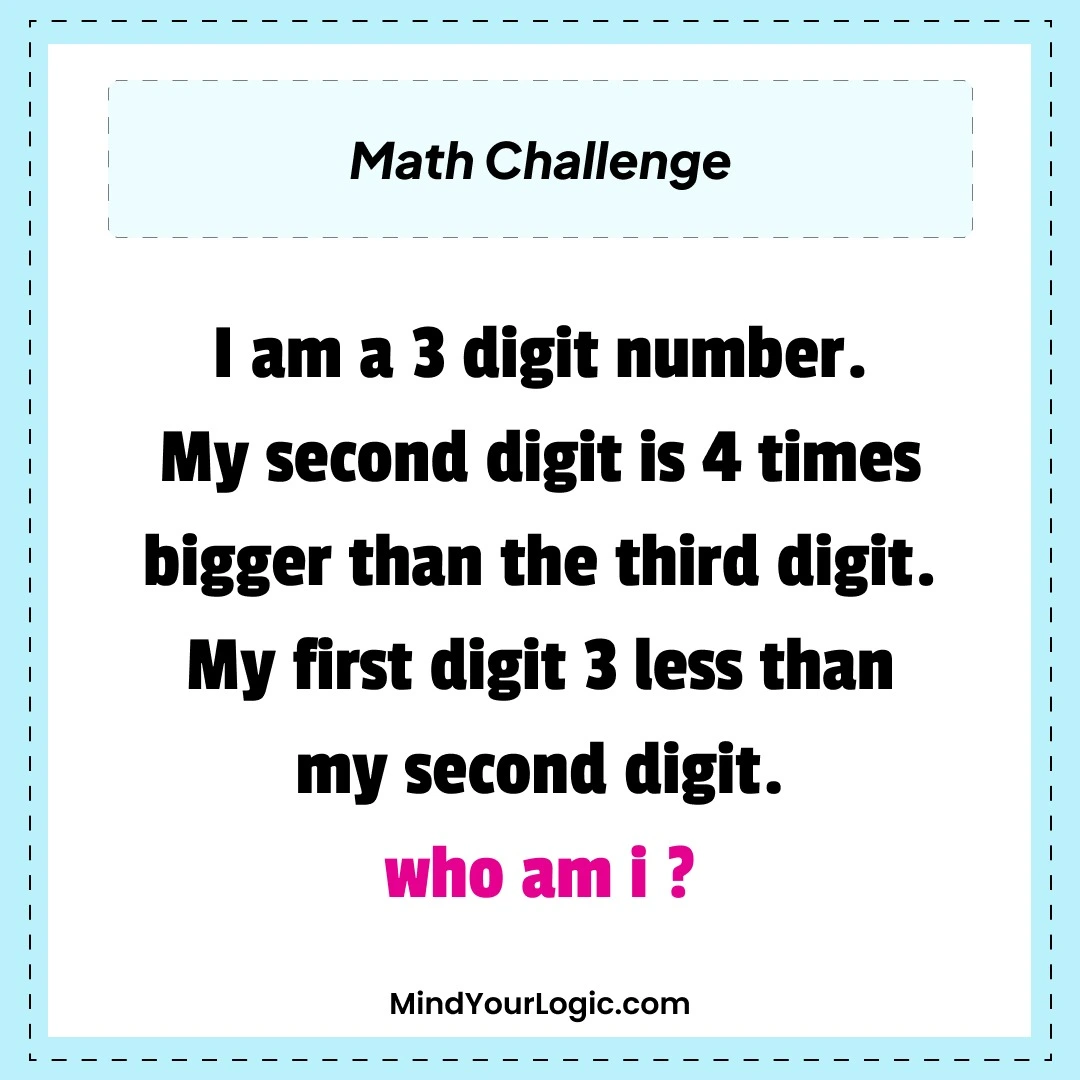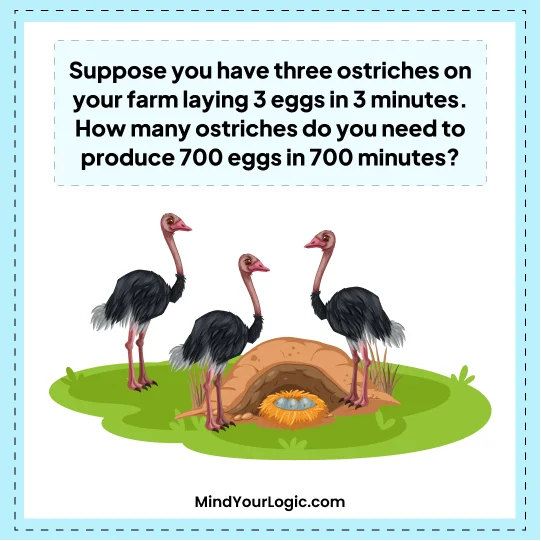March 11, 2023

# Can you solve this equation (3∆5)∆2=?

If ∆ means multiplying the first number by the second, then (3∆5)∆2 =?• A. 40
• B. 35
• C. 30
• D. None of these

Explanation :

we need to multiply the first number (3) by the alternate number (5). thus, (3∆5) is equal to 3 multiplied by 5, which equals 15. Next, we've to calculate(15∆2) using the same note.

Again, we multiply the first number (15) by the alternate number (2) to get the result. thus,(15∆2) is equal to 15 multiplied by 2, which equals 30. thus, the answer is 30. We can represent the entire expression using the accurate note as follows (3∆5)∆2 = (3x5)∆2 = 15∆2 = 15 x 2 = 30 So, the final result of the expression(3∆5)∆2 using the given note is 30.

## Solve More Riddles##### If A is substituted by 4,B by 3 | Math Puzzle

If A is substituted by 4, B by 3, C by 2, D by 4, E by 3, F by 2 and so on, then what will be the to ...##### There's A Three Digit Number Math Puzzle

I am a three-digit number my second digit is four times bigger than the third digit my first digit 3 ...##### How Many Eggs Ostriches Lay in 700 Minutes? Math Riddle

Suppose you have three ostriches on your farm laying three eggs in three minutes. How many ostriches ...